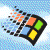Carb's Computer Site# Binary

Many people know that binary is "Zeroes and ones" and many know that it has something to do with computers, but what and why? How does it make any sense?

Binary in one sense is no more than a numbering system used to represent (almost) any number imaginable. We naturally represent numbers with a system called "Decimal" which uses ten unique symbols to represent our numbers (1, 2, 3, 4, 5, 6, 7, 8, 9, 0) and in contrast, binary only uses two symbols (1, 0). You might already know or have realised that "dec" means "ten" and "bi" means "two", which helps us make a lot more sense of these names (examples of other named numbering systems might include trinary with 3 unique symbols or hexadecimal which has sixteen), and a key difference between these two numbering systems is that a single symbol in decimal is often called a "digit" whereas in binary it is known as a "bit". The reason binary is more useful than decimal in certain contexts is that it maps easily onto numbers as well as simple signals: on and off! Once you learn how binary works, many other strange things about computers may click into place.

Basic binary (whole, positive numbers) is the simplest place to begin. Starting from the right hand side, each one or zero represents an exponent of 2 (as in, 2 to the power of X where X is the position of the bit in the sequence). 1 is "Two to the power of zero". 10 is "Two to the power of one" and 100 is "Two to the power of two". The values of each bit are then added up, so the previous examples alone are equivalent to 1, 2 and 4 respectively, meaning 111 would be 7 (1 + 2 + 4 = 7)! How do we know that 110100010110 is equal to 3,350? How do we work that out? Let's use a table to make this easier:

 1 1 0 1 0 0 0 1 0 1 1 0 2^11 2^10 2^9 2^8 2^7 2^6 2^5 2^4 2^3 2^2 2^1 2^0

And if we work out what those exponents mean...

 1 1 0 1 0 0 0 1 0 1 1 0 2048 1024 512 256 128 64 32 16 8 4 2 1

Ok cool, let's ignore the ones where the bit is "off" or in other words, zero (another way to think of this is that the sum of each bit is the exponent value multiplied by the bit. 1024 * 1 = 1024. 512 * 0 = 0.

 1 1 0 1 0 0 0 1 0 1 1 0 2048 1024 0 256 0 0 0 16 0 4 2 1

This means that the final value is 2048 + 1024 + 256 + 16 + 4 + 2.... That's 3350!

If you don't want to get lost in the "exponents", you can just skip to working things out in a manner similar to the second table. It's easy to keep multiplying by 2 to get the numbers (1, 2, 4, 8...) and simplifies the whole thing by being less math-y. If you want to work anything out in binary, I completely recommend writing it out in a grid like above!

Binary math can be quite simple. Addition works by combining the two binary numbers and "carrying the one" where appropriate, probably just like you learned in school (except you don't carry the one when you reach ten, you do it when you reach two). Here's some examples of binary additions to help you understand, with their equivalent in decimal:

• 1 + 10 = 11 (1 + 2 = 3)
• 101 + 10 = 111 (5 + 2 = 7)
• 10 + 10 = 100 (2 + 2 = 4)
• 1111 + 1111 = 11110 (15 + 15 = 30)
• 0 + 1 = 1 (0 + 1 = 1)

This "combine the bits and carry over" tactic translates really well to electronics. So well in fact that sometimes multiplication is much easier electronically represented as multiple additions!